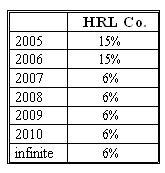### Why should I choose AnalystNotes?

Simply put: AnalystNotes offers the best value and the best product available to help you pass your exams.

##### Subject 8. Estimating and Calculating the Implied Expected Rate of Return
Assuming that the markets are efficient, we use DDM models for calculation of the expected rate of return on a stock. In some cases, such as the H-model, we can directly express the expected rate of return as a function of market price and forecasted dividends.

For H-model, the implied rate of return is

r = D0 /P0 [(1 + gL) + H (gS - gL)] + gL

Note that if gL = gS the expression becomes identical to the Gordon growth model.

Other models, such as the general two-stage DDM, three-stage DDM and more complicated spreadsheet models can be solved for the effective rate of return only by iteration. We change the discount rate until the present value of dividends and the terminal value is equal to the market price. If we believe that the market price differs from the intrinsic value of a stock, we need to adjust the expected required rate of return accordingly. This is the same approach that is used for the calculation of IRR in corporate finance.

Example

Beth is evaluating HRL Co. which has been growing at the annual rate of 15% for the last 5 years. After considerable study, she has decided to use the following information in her valuation (as of beginning of 2005):

• 2004 dividend: \$5.
• The current market price of HRL: \$120.

Here is her forecast of the company's future dividend growth trend.What is the required rate of return implied in the market?

P = D1 / (1 + r)1 + D2 / (1 + r)2 + V2 / (1 + r)2

P = 120, D1 = 5 x 1.15 = 5.75, D2 = 5 x 1.152 = 6.61, V2 = 6.61 x 1.06 / (r - 0.06). Consequently, 120 = 5.75 / (1 + r) + 6.61 / (1 + r)2 + 7.01 / [(1 + r)2 (r - 0.06)]

Iterating different interest rates into the equation we can find that the expected rate of return is close to 11.2%.

Learning Outcome Statements

estimate a required return based on any DDM, including the Gordon growth model and the H-model;

CFA® 2023 Level II Curriculum, Volume 3, Module 23